• public class Testcon {  private int num;     public Testcon(int num){      this.num=num;     }     public int getNum(){ ...&nbs...
public class Testcon {  private int num;     public Testcon(int num){      this.num=num;     }     public int getNum(){      return num;     }     public void setNum(int temp){      this.num=temp;     }     //交換類     public static void swap(Testcon a,Testcon b){      int temp=a.getNum();       a.setNum(b.getNum());          b.setNum(temp);     }         public static void main(String[] args){      Testcon a=new Testcon(25);      Testcon b=new Testcon(50);         System.out.println(“交换前a,b的值为：”+a.getNum()+","+b.getNum());         swap(a,b);         System.out.println(“交换后a,b的值为：”+a.getNum()+","+b.getNum());       } }
展开全文• 1.1：值传参方法 //值传参 void NoChangeValue(int v) { printf("值传参修改前的值 = %d\n", v); v = 10; printf("值传参修改后的值 = %d\n", v); } 1.2：主方法 void main() { int n = 1; printf("\n--------...
1、值传参，对形参的任何操作都不会对实参产生影响
1.1：值传参方法
//值传参
void NoChangeValue(int v) {
printf("值传参修改前的值 = %d\n", v);
v = 10;
printf("值传参修改后的值 = %d\n", v);
}

1.2：主方法
void main() {
int n = 1;
printf("\n----------------------NoChangeValue------------------------------------\n\n");
printf("调用前的值是 = %d\n", n);
printf("\n------------start---------\n\n");
NoChangeValue(n);
printf("\n------------end-----------\n\n");
printf("调用后的值 = %d,还是原值\n", n);
}

1.3：运行结果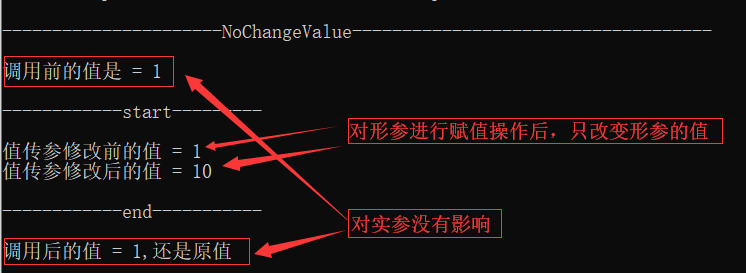1.4：解释
当主程序调用一个方法时，会产生一个栈帧。在栈帧中，包含方法的形参、方法的局部变量。执行方法时，会将实参的值赋值给栈帧中的形参。因此：对形参的操作不会影响到实参。
2、值的引用传参，对形参的任何操作都会对实参产生影响
2.1：引用传参方法
void ChangeValue(int &v) {
printf("值传参修改前的值 = %d\n", v);
v = 10;
printf("值传参修改后的值 = %d\n", v);
}

2.2：主方法
void main(){
int n = 2;
int* p = &n;
printf("\n----------------------ChangeValue------------------------------------\n\n");
printf("调用前的值是 = %d\n", n);
printf("\n------------start---------\n\n");
ChangeValue(n);
printf("\n------------end-----------\n\n");
printf("调用后的值是 = %d，不再是原值\n", n);
}

2.3：运行结果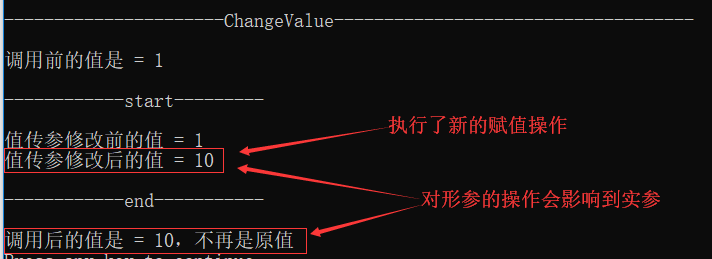2.4：解释
引用相当于是外部变量的别名，实际操作的就是该变量，即在函数内对该变量进行修改的话，在外部该变量也会相应被修改。
3、指针传参第一种，直接改变指向的值
3.1：指针传参方法
void ChangePtrValue(int* p){
printf("分配内存前p的地址 = %d \t 分配内存前p指向的地址 = %d \t 分配内存前p指向地址上的数值 = %d\t\n", &p,p,*p);
*p = 3;//直接改变指向的值
printf("分配内存后p的地址 = %d \t 分配内存后p指向的地址 = %d \t 分配内存后p指向地址上的数值 = %d\t\n", &p,p,*p);
}

3.2：主方法
void main(){
int n = 2;
int* p = &n;
printf("----------------------ChangePtrValue------------------------------------\n\n");
printf("方法调用前p的地址 = %d \t 方法调用前p指向的地址 = %d \t 方法调用前p指向地址上的数值 = %d\t\n", &p,p,*p);
printf("\n------------start---------\n\n");
ChangePtrValue(p);
printf("\n------------end-----------\n\n");
printf("方法调用后p的地址 = %d \t 方法调用后p指向的地址 = %d \t 方法调用后p指向地址上的数值 = %d\t\n", &p,p,*p);
}

3.3：运行结果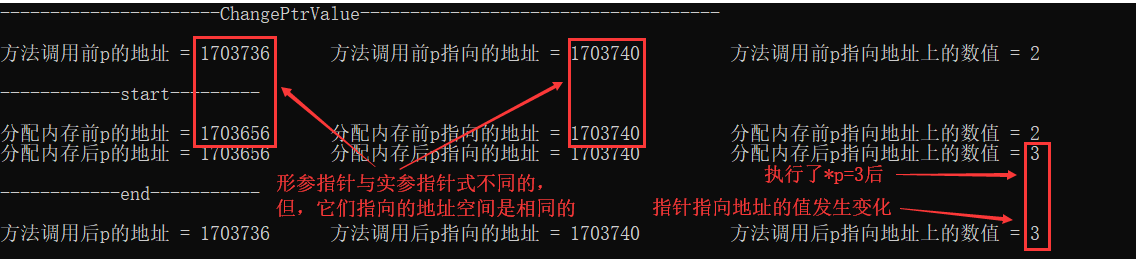3.4：解释
第一种是直接把值存放到指针存放的地址空间中，即：*p=10
4、指针传参第二种，通过改变指针指向的地址空间，来间接的改变指针指向的值（其实并不可以改变）
4.1：指针传参方法
//不可改变指针所指向的地址
printf("分配内存前p的地址 = %d \t 分配内存前p指向的地址 = %d \t 分配内存前p指向地址上的数值 = %d\t\n", &p,p,*p);
int n = 3;
p = &n;
printf("分配内存后p的地址 = %d \t 分配内存后p指向的地址 = %d \t 分配内存后p指向地址上的数值 = %d\t\n", &p,p,*p);
}

4.2：主方法
void main(){
int n = 2;
int* p = &n;
printf("方法调用前p的地址 = %d \t 方法调用前p指向的地址 = %d \t 方法调用前p指向地址上的数值 = %d\t\n", &p,p,*p);
printf("\n------------start---------\n\n");
printf("\n------------end-----------\n\n");
printf("方法调用后p的地址 = %d \t 方法调用后p指向的地址 = %d \t 方法调用后p指向地址上的数值 = %d\t\n", &p,p,*p);
}

4.3：运行结果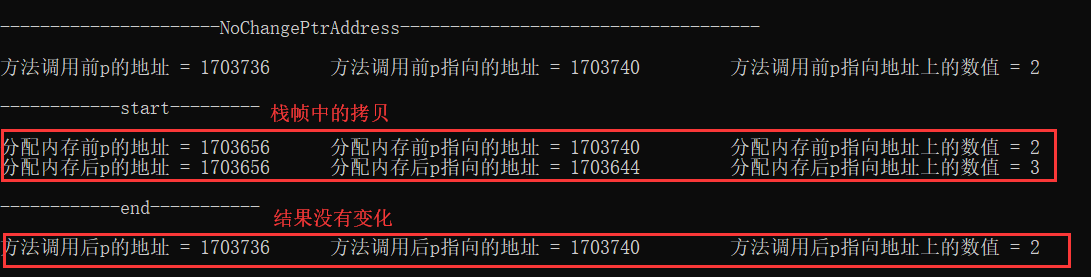4.4：解释
第二种是在方法中通过将变量的地址存放到形参指针中，使形参指针指向另一个地址空间，间接的修改形参指针指向的值。在方法调用过程生成的栈帧中，该指针是局部的，是因为它只是实参指针的一个拷贝，对局部变量 的修改并不会改变实际指针。因此：不会像指针传参第一种一样。
5、指针引用传参第一类，仅会改变指针指向的地址（即改变指针的内容），而使得指针指向的值出现非法现象
5.1：指针传参方法
//可改变指针所指向的地址
printf("分配内存前p的地址 = %d \t 分配内存前p指向的地址 = %d \t 分配内存前p指向地址上的数值 = %d\t\n", &p,p,*p);
int n = 3;
p = &n;//改变指针指向的地址空间
printf("分配内存后p的地址 = %d \t 分配内存后p指向的地址 = %d \t 分配内存后p指向地址上的数值 = %d\t\n", &p,p,*p);
}

5.2：主方法
void main(){
int n = 2;
int* p = &n;
printf("方法调用前p的地址 = %d \t 方法调用前p指向的地址 = %d \t 方法调用前p指向地址上的数值 = %d\t\n", &p,p,*p);
printf("\n------------start---------\n\n");
printf("\n------------end-----------\n\n");
printf("方法调用后p的地址 = %d \t 方法调用后p指向的地址 = %d \t 方法调用后p指向地址上的数值 = %d\t\n", &p,p,*p);
}

5.3：运行结果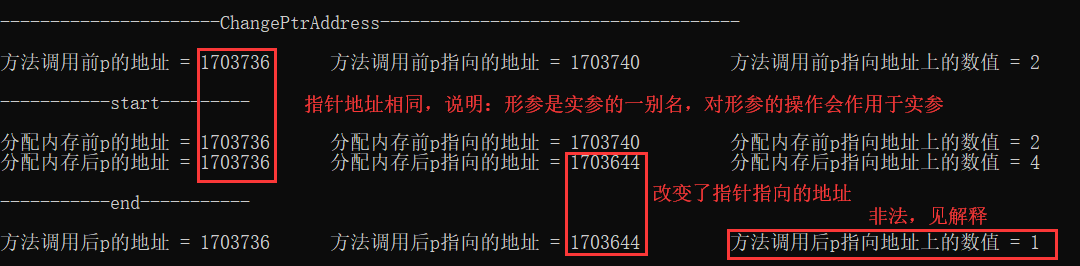5.4：解释
之所有会出现非法，是因为int n = 3；p = &n；n是一个局部变量，当方法调用结束时，会对该空间进行释放，因此出现不符合实际的情况。而指针指向的地址发生改变（虽然该地址不存在），这是因为在引用传参中，对形参的操作就是对实参的操作。
6、指针引用传参第二类，如同指针传参第一种，直接改变指向的值(即使用*p=10;)。再在此不在多讲。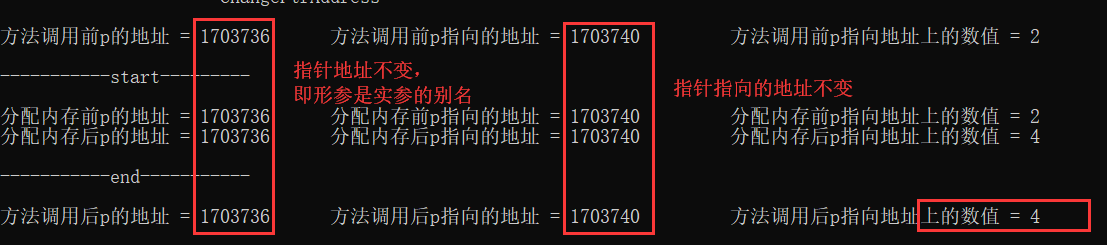7、结论
若要改变值实参，通过值引用传参、或通过指向其地址的指针来进行指针传参。 若要改变指针中的内容（即指针指向的地址），使用指针引用传参第一类。 若要改变指针指向地址空间的内容，使用指针引用传参第二类或指针传参第一类。
注：本人在二叉树初始化时，由于没有使用指针的引用传参，出现二叉树初始化失败，指针返回的结果老是为NULL。
展开全文指针 实参与形参
• 在C++中，引用的表示方法与指针相似，只是用&amp;代替了*。引用（reference）是c++对c语言的重要扩充。引用就是某一变量（目标）的一个别名，对引用的操作与对变量直接操作完全一样。引用的声明方法：类型标识符...

在C++中，引用的表示方法与指针相似，只是用&代替了*。引用（reference）是c++对c语言的重要扩充。引用就是某一变量（目标）的一个别名，对引用的操作与对变量直接操作完全一样。引用的声明方法：类型标识符 &引用名=目标变量名；
引用作为变量的别名，在一些场合可以代替指针；
引用相对指针来说有更好的可读性和实用性；
引用本质是对象的一个常指针
void function(int &a)
{
a=100;
}
//引用转化为指针的函数
void function(int * const a)
{
*a=100;
}

1. 单独定义引用时，必须要初始化；
int &a;//错误; 引用变量 "a" 需要初始值设定
2. 改变引用变量的值，同时也会改变原变量
int a = 0;
int &b = a;
b = 100;
cout << a;//输出：100
3.函数返回“非静态”栈变量的引用，栈空间会被回收，不能作为其它变量的初始值
int getVal1()//返回值本身
{
int a = 100;
return a;
}
int& getVal2()//返回引用
{
int a = 100;
return a;
}
int* getVal3()//返回指针
{
int a = 100;
return &a;
}

int main()
{
int a1 = getVal1();
int a2 = getVal2();//使用int去接收,打印：100
int &a22 = getVal2();//使用引用接收，打印：随机地址值
int *a3 = getVal3();//同a22
return 0;
}

4.函数返回“静态”栈变量引用，输出正常；因为static修饰的变量，存放在静态存储区，具备持久性；
int getVal1()//返回值本身
{
static int a = 100;//static 修饰的局部变量；注意：Java不支持这种写法；
return a;
}
int& getVal2()//返回引用
{
static int a = 100;
return a;
}
int* getVal3()//返回指针
{
static int a = 100;
return &a;
}

int main()
{
int a1 = getVal1();
int a2 = getVal2();//使用int去接收,打印：100
int &a22 = getVal2();//使用引用接收，打印：100
int *a3 = getVal3();//同a22
return 0;
}

5.基本数据类型传参
//交换了拷贝的值，不能交换实参本身的值; 与Java中效果一样
void swap0(int a, int b)
{
int c = a;
a = b;
b = c;
}
void swap(int& a, int & b)
{
int c = a;
a = b;
b = c;
}
void swap(int* a, int * b)
{
int c = *a;//注意： 不能定义为 int *c=a;去交换
*a = *b;
*b = c;
}

int main()
{
int a = 1, b = 2;
printf("swap1交换前,a:%d,b:%d\n", a, b);
swap1(a,b);
printf("swap1交换之后,a:%d,b:%d\n",a,b);

printf("swap2交换前,a:%d,b:%d\n", a, b);
swap2(a, b);
printf("swap2交换之后,a:%d,b:%d\n", a, b);

printf("swap3交换前,a:%d,b:%d\n", a, b);
swap3(&a, &b);
printf("swap3交换之后,a:%d,b:%d\n", a, b);
}
//输出
swap1交换前,a:1,b:2
swap1交换之后,a:1,b:2
swap2交换前,a:1,b:2
swap2交换之后,a:2,b:1
swap3交换前,a:2,b:1
swap3交换之后,a:1,b:2

6. 复杂数据类型传参
在Java中：
    static class Person{
private int age;
public Person(){}
public Person(int age){
this.age=age;
}
public int getAge() {
return age;
}
public void setAge(int age) {
this.age = age;
}
}
public static void edit1(Person p){
p.setAge(100);
}
public static void main(String args[]){
Person p1=new Person(11);
System.out.println("edit之前,p1:"+p1.getAge());
edit1(p1);
System.out.println("edit之后,p1:"+p1.getAge());
}
输出：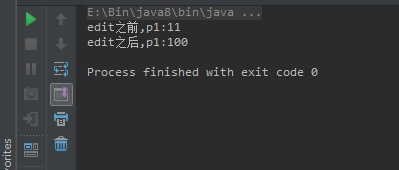=>对象的形参与实参指向同一块内存；

在C++中：
#include <iostream>
using namespace std;

class Person {
private:
int age;
public:
int getAge() {
return age;
}
void setAge(int age) {
this->age = age;
}
Person() {}
Person(int age) {
this->age = age;
}
};
void edit1(Person p) {
p.setAge(100);
}
void edit2(Person &p) {
p.setAge(100);
}
void edit3(Person *p) {
p->setAge(100);
}
int main()
{

Person p1(11);
printf("edit1之前,p1:%d\n", p1.getAge());
edit1(p1);
printf("edit1之后,p1:%d\n", p1.getAge());

p1.setAge(11);
printf("edit2之前,p1:%d\n", p1.getAge());
edit2(p1);
printf("edit2之后,p1:%d\n", p1.getAge());

p1.setAge(11);
printf("edit3之前,p1:%d\n", p1.getAge());
edit3(&p1);
printf("edit3之后,p1:%d\n", p1.getAge());

system("pause");
return 0;
}输出：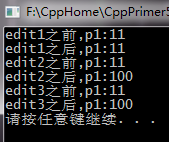可以看出edit1,类似Java的写法，在Java中可以更改p1对象的值，在c++中无效；
原因：
*  C++中声明对象 Person p; 会申请一块新内存，去初始化Person对象；
* Java中声明对象 Person p; 只声明不赋值，则只会在内存的栈区创建对象的引用，并没有在堆区申请对象的空间；
=> C++中的引用传递类似Java中的复杂对象的传递方式；

展开全文• 方法调用传参按照数据类型分为两种： 数据类型分为两大类： 基本数据类型和引用数据类型（类，接口，数组） 一、基本数据类型传参 例： public class Test { public static void main(String[] args) { //交换两个...
方法调用传参按照数据类型分为两种： 数据类型分为两大类： 基本数据类型和引用数据类型（类，接口，数组） 一、基本数据类型传参 例：
public class Test {
public static void main(String[] args) {
//交换两个变量的值
int num0 = 10;
int num1 = 20;
System.out.println("num0=" + num0 + "\tnum1=" + num1);
change(num0, num1);
System.out.println("num0=" + num0 + "\tnum1=" + num1);
}

static void change(int a, int b) {
System.out.println("a=" + a + "\tb=" + b);
int temp = a;
a = b;
b = temp;
System.out.println("a=" + a + "\tb=" + b);
}
}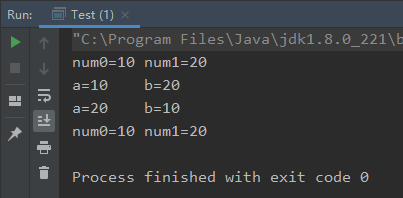内存分析：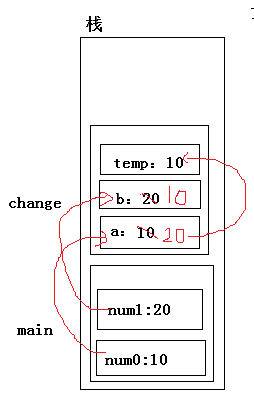任何一个方法当被调用时，会生成一个对应方法的栈帧，每个方法的栈帧中保存的是方法内的局部变量的内容。当方法结束时，对应的栈帧被移除。 小结：开辟对应的方法的栈帧，给所有的形式参数开辟内存，将实参的值赋值给形式参数。 即给形参分配内存，并将实参的值赋给形参。（简言之，基本数据类型传参，传递的是实参的值，并将实参的值夫给形参。做的是值传递，即pass by value）; 二、引用数据类型传参（引用数据类型作为方法的参数） 与基本数据类型相同，都会给形参开辟内存，但是不同的是实参传递给形参的是实参的引用，而不是实参的值。 例：
public class Test {
public static void main(String[] args) {
//交换两个变量的值
int []arr={1,2};
System.out.println("num0="+arr+"\tnum1="+arr);
change(arr);
System.out.println("num0="+arr+"\tnum1="+arr);
}
static void change(int[] arr) {
int temp = arr;
arr=arr;
arr=temp;
}
}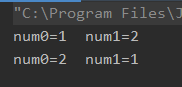内存分析：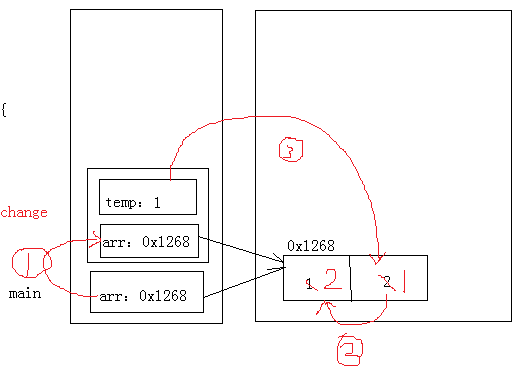小结：传引用（pass by reference）
展开全文• 引用符号&...虽然引用和指针的方式作为传参方式都会保留该函数对传入参数的改变，但这两种方法还是有区别的。这个区别表现在，引用的方式下可以直接使用该参数，而指针的方式通常都需要解引用，或c++
• 在Java方法传参中有一个让疑惑又很经典的问题：在方法传递时，到底传递的是值，还是引用？ 其实Java只有值传递这一种。不过是传数据值还是地址值的问题。 首先我们要知道两个Java存储区：栈（虚拟机栈）和堆。 我们...
• 理解了new关键字创建对象的过程，再来看看引用传参。Java中的引用一共有三种，数组、类对象、接口，下面着重看看数组和类对象的传参过程。 [java] view plain copy public class Test {   public ...
• 1.1 代码实例 package st; public class Apple { public static void yinYong...引用传参。&amp;amp;quot;); System.out.println(&amp;amp;quot;yinYong里面的str:&amp;amp;quot; + &amp;amp;quot;
• java小记：Java中String引用传参我们知道在java中字符串（Stirng）对象和其他对象不太一样，是不可变的，所以再作为参数传递的时候和基本类型一样，都是“值传参”，要想达到“引用传参”的效果，就要使用...java String
• 3种传参方式：值传参、指针传参、引用传参我们知道传参方式有3种：值传参、指针传参、引用传参。这三种方式书写方式各有不同，接下来，我们简单介绍3种传参方式实现的机制。首先看一个程序：这个程序实现的是交换2个...
• 引用 ：真正的以地址的方式传递参数传递以后，行参和实参都是同一个对象，只是他们名字不同而已对行参的修改将影响实参的值 说明： 传值：根copy是一样的。打个比方，我有一橦房子，我给你建筑材料，你建了...
• 4、使用引用传参的原因 1、直接传参 直接传参是直接开辟了一个跟主函数实参一样的空间（地址不一样），里面存放了了跟实参一样大小的值，就相当于数值大小相同但是位置不同。在这个调用函数里使用这个一样大小...c语言
• 通过引用类型进行方法传参需要知道如下几点： 当参数是基本数据类型时，方法不能修改实际参数的值。 当参数是引用类型时，方法通过地址引用改变了实际参数对象的内容。 基本引用类型有：类、接口、数组。 以下...
• ## java方法间传参问题

千次阅读 2019-03-05 11:00:15
java方法传参问题   有些人会迷惑java方法传参的时候有时候会改变原来的值，有时候不会，这是为什么呢？其实这是要分很多种情况的：原始类型，引用类型，不可变类型，改变值，改变地址。   看一些简单的例子 ...java
• Java 流式操作 Stream(15)面向对象引用传参&&函数式引用传参 package com.lius.stream; import java.util.function.Consumer; import java.util.function.IntConsumer; /** * <p>面向对象引用...stream lambda java
• ## 方法以及方法传参

千次阅读 2018-05-17 15:26:16
方法中有无参方法和带参方法两种，无参方法 没有参数，可以有返回值也可以没有返回值。有返回值的要有返回值的类型（既数据类型）。 没有返回值的用void修饰。带参方法 带参方法就是方法定义处带有参数，且定义了...
• 所遇问题 最近在做项目遇到了一个问题，具体场景是多线程下共享资源的问题，这里模拟一个具体场景： 有一个启动线程的类，这个类中存放线程的共享变量，在这个类中新建多个线程，并将共享变量以构造器的参数引入，...
• NULL 博文链接：https://m635674608.iteye.com/blog/1513061
• 这段代码的输出值是goodandgbc很明显，传入的String没有被改变，而传入的char数组的值却被改变了。首先对于传参来说，区别在在于数据类型， 一、对于基本数据类型来说是不能修改基本...二、对于对象引用类型的传参对于java
• 由于引用被设计为对象的别名，所以它被定义后不能再指向其它对象，它也不能指向空对象 对引用进行的操作，和对真实对象进行操作，效果是完全一样的，包括取值运算 int main() { int a = 25; int &r = a; ...
• 可以使用“&”符号设定为引用传递，形式如下： function f1( $p1, &$p2, .... ）{ 。。。。。 } 此时，在函数内部，对该形参变量改变其值，则对应的实参变量（在函数外部）的值也改变了。
• 但是传参的时候传入的是一个指向链表节点指针的引用类型 也就是说在其中打***的地方  不会因为没有把前面的节点链接到后面而导致断链 相反 这里的l = l->next 是把当前节点的地址指向了后面 比如对于1 3 1...
• Gender.cs /// <summary> /// 性别类型 /// <...//枚举中不能定义字段属性和方法 //枚举值是从0递增的整数 Child.cs using System; using System.Collections.Generic; using System...
• 如果一个方法传入的对象没有new一个新的对象就是引用传参 new一个新的对象就不是引用传参了 C：总结 1. 因此可见： 在Java中所有的参数传递，不管基本类型还是引用类型， 都是值传递，或者说是副本传递。 只是在...
• ◇在Java中不能用按引用调用或按指针调用。不管传递给方法的实际参数是什么类型， 相关的形式参数都会得到该数据的一份拷贝，这就是按值调用的工作原理。 ◇局部变量在调用栈中分配内存，当方法执行完成后，...
• -- 把传递过来的参数进行页面渲染 --> 我是引用模板中的文字的小憨憨</text> </view> </template> <script> export default { data() { return { hello:'我才有一个参数需要你进行接受' }; } }; ...vue js vue.js
• 当我想通过change方法改变node时 测试方法： ` public static void main(String[] args) { Test test=new Test(); System.out.println(test.node); test.go(); System.out.println(test.node); } ...java java-ee
• @Description: 方法传参 */ public class TestCon { public static void swap(MyTest a, MyTest b) { int tmp = a.getNum(); a.setNum(b.getNum()); b.setNum(tmp); } public static void main...
• 基本数据类型 整型：byte，short，int，long浮点型：float，double字符型：char布尔型：boolean引用数据类型 数组类接口方法的参数分为实际参数，和形式参数。形式参数：定义方法时写的参数。实际参数：调用方法时...JAVA...